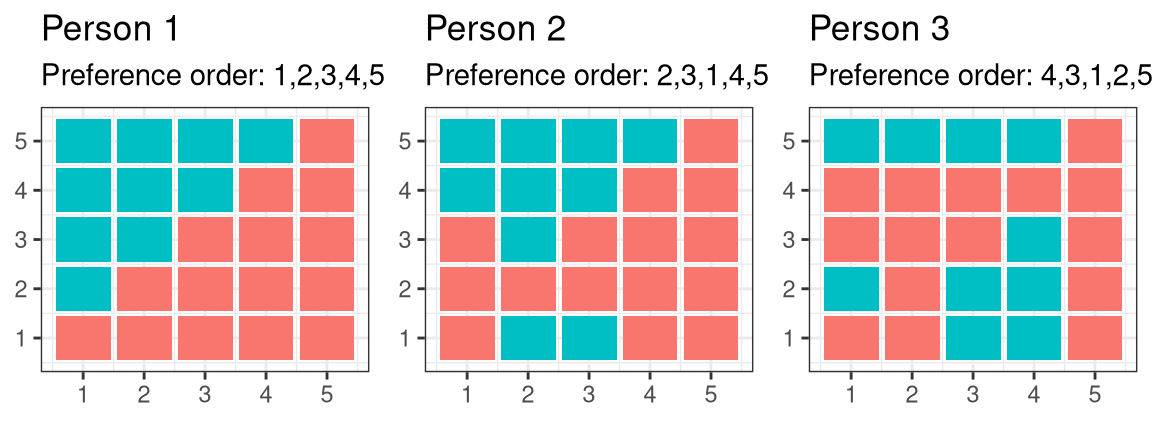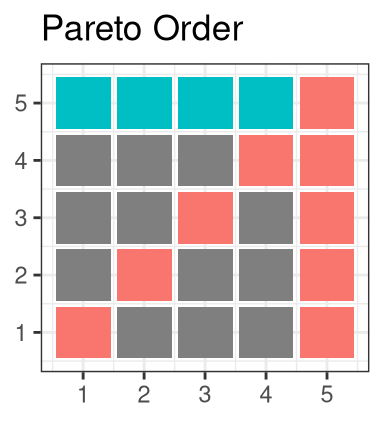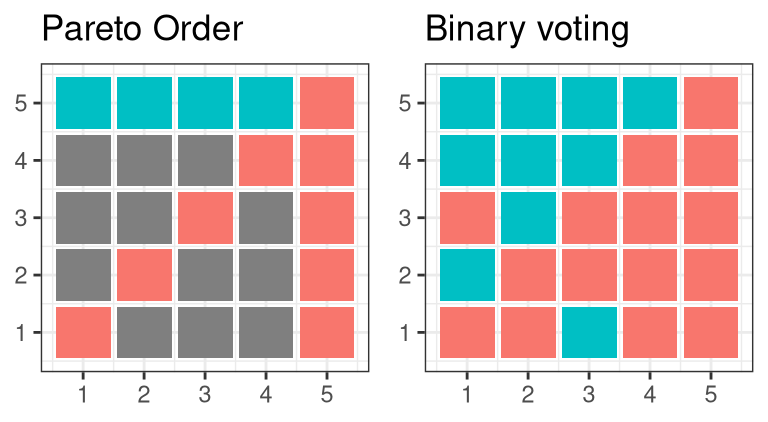# Sets

## Definition & Notation

• Well-specified collection of elements
• set $$A$$, element $$a \in A$$
• Example: $A = \{4, 5, 6 \} = \{ n \in \mathbb{N} : 3 < n < 7 \}$

## Operations & relations

• Union: $$A \cup B = \{x: x\in A \text{ or } x \in B\}$$.
• Intersect: $$A \cap B = \{x: x \in A \text{ and } x \in B\}$$.
• Minus: $$A \setminus B = \{ x: x\in A \text{ and } \not\in B \}$$
• Product: $$A \times B = \{(x,y): x \in A, y \in B \}$$
• Power set: $$\mathcal{P}(A) =$$ set of all subsets of $$A$$
• Subset $$A \subseteq B$$ means that if $$a\in A$$ then $$a \in B$$
• $$A \subset B$$ means $$A \subseteq B$$ and $$\exists b \in B$$ such that $$b \not\in A$$

# Relations

## Relations

• relation on two sets $$A$$ and $$B$$ is any subset of $$A \times B$$, $$R \subseteq A \times B$$. We usually denote relations by $$a \sim_R b$$ if $$(a,b) \in R$$
• Example: $$A=B=\mathbb{R}$$, $$<$$ is associated with $$R_{<} = \{(a,b) \in \mathbb{R}^2 : a<b \}$$

## Equivalence Relation

• equivalence relation is
• relexive: $$a \sim_R a$$ $$\forall a \in A$$
• transitive: $$a \sim_R b$$ and $$b \sim_R c$$ implies $$a \sim_R c$$
• symmetric: $$a \sim_R b$$ implies $$b \sim_R a$$,
• partitions $$A$$ into equivalence classes, $$\sim(x) = \{a \in A: a \sim x \}$$

## Weak order

• $$\preceq$$ is a weak order if
• relexive: $$a \preceq a$$ $$\forall a \in A$$
• transitive: $$a \preceq b$$ and $$b \preceq c$$ implies $a$c
• complete: for any $$a, b \in A$$, either $$a \preceq b$$ or $$b \preceq a$$ (or both)
• Example: preference relation

## Pareto order

• $$n$$ individuals, preferences $$\succeq_i$$
• Pareto order: $x \succeq^P y \text{ if } x \succeq_i y \text{ for all } i=1,...,n$
• transitive? reflexive? complete?## Social choice rule

• Combines preferences into single weak order $$F(\succeq_1, ..., \succeq_n) = \succeq$$
• Completes Pareto order if $$x \succeq^P y$$ implies $$x \succeq y$$

## Examples

• Binary voting
• social choice rule is determined by majority vote on each pair $x \succeq y \iff x \succeq_i y \text{ for at least (n+1)/2 individuals }$## Examples

• Majority voting
• Each person votes for most preferred outcome
• Social choice rule ranks by number of votes
• Ties broken by number of votes for second most preferred, then third, etc